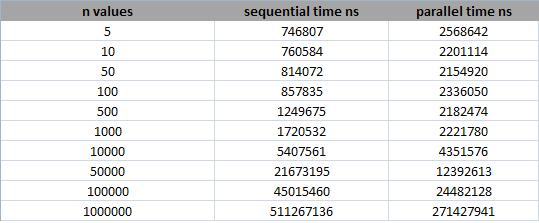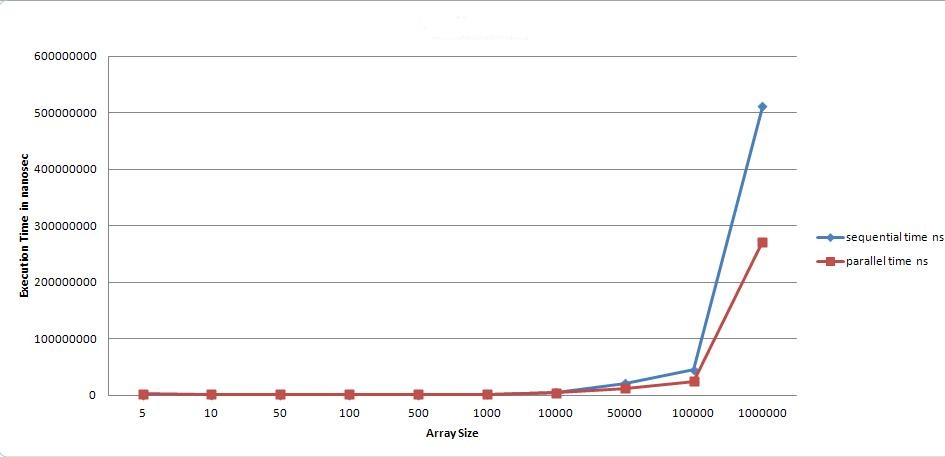Sharing Our Passion for Technology
& continuous learning

### Parallel Programming With Barrier Synchronization

Parallel Programming is an emerging computer science field that studies the opportunity of splitting data into small chucks and process them on multiple processors simultaneously which provides a faster execution time. Parallel programming is useful in sorting, image processing, network processing and may other memory intensive tasks.

For parallel program execution to be effective and fruitful, we need to run programs on computers with multiple CPUs or cores. Of course, most people have computers with multiple processors and they are interested in high speed execution. This opens the way for parallel programming.

Having a sequential program running on a machine with multiple processors does not mean it will run faster than on a single processor computer. But why? Let’s say we have five processors on a machine, a running sequential program would leave four CPUs untouched and run on only one processor. This will execute in the same amount of time it would take on a single processor machine. On the other hand, a parallel program designed to run on multiple processors can perform the same task in less time.

Java provides parallel programming capabilities with different frameworks and APIs. This example is using CyclicBarrier. We have two programs, one is sequential and the other is parallel. Both of them have the same input and should give the same output. The Algorithm I’m using is a merge sort. In both cases they take two different sorted lists and merges them into new sorted list which has a size equal to the sum of their sizes.

For the parallel version I’m using a Barrier Synchronization algorithm to parallelize the merge sort.

Example:-

Before merge: x = [1,3,5,7,9] y = [2,4,6,8,10] z = [0,0,0,0,0,0,0,0,0,0]

After merge: x = [1,3,5,7,9] y = [2,4,6,8,10] z = [1,2,3,4,5,6,7,8,9,10]

I created a method that takes x, y and z and called merge, this method compares list y with list x and replace the element of y in the proper positions of list z sorted as follows: merge(x,y,z) change the value of z to be (z = [0,2,0,4,0,6,0,8,0,10]) Then we apply the merge method with changing x and y places in the method call merge(y,x,z) change the value of z to be (z = [1,2,3,4,5,6,7,8,9,10])

Now let’s take a look at the code: Merger.java

``````package com.sourceallies.test.sort;

/**
* Utility class that is used for merging two lists in one list
*
*
*/
public class Merger {
/**
* Method that compare y elements with x and puts them in the proper place in
* z
*
* @param x
* @param y
* @param z
*/
public static void merge(int[] x, int[] y, int[] z) {
for (int i = 0; i &lt; y.length; i++) {
int low = 0;
int mid = 0;
int high = y.length;
int val = y[i];
while (low &lt; high) {
mid = low + ((high - low) / 2);
if (x[mid] &lt; val)
low = mid + 1;
else
high = mid;
}
z[i + low] = val;
}
}
}
``````

``````package com.sourceallies.test.sort;

import java.util.concurrent.BrokenBarrierException;
import java.util.concurrent.CyclicBarrier;

public class ThreadX implements Runnable {
int[] x;
int[] y;
int[] z;
CyclicBarrier barrier;
/**
* Constructor that passes the arrays and the shared barrier to the thread.
*
* @param x
* @param y
* @param z
* @param barrier
*/
public ThreadX(int[] x, int[] y, int[] z, CyclicBarrier barrier) {
super();
this.x = x;
this.y = y;
this.z = z;
this.barrier = barrier;
}

/**
*
*/
@Override
public void run() {
handle(x, y, z, barrier);
}

/**
* call the merge method with barriers,
* each thread will wait for the other threads on that barrier and until all of them are done,
* then they will leave the barrier after that in a sync way
*
*/
private void handle(int[] x, int[] y, int[] z, CyclicBarrier barrier) {
try {
Merger.merge(x, y, z);
//force the thread to wait on the barrier.
barrier.await();
} catch (InterruptedException e) {
e.printStackTrace();
} catch (BrokenBarrierException e) {
e.printStackTrace();
}
}
}
``````

ParallelApplication.java

``````package com.sourceallies.test.sort;

import java.util.concurrent.CyclicBarrier;

/**
* Class that is used to pass the two arrays x, y into two Threads that will
* merge sort them in array z then calculate the elapsed time and show it on the
* console
*
*
*/
public class ParallelApplication {

public static void test(int[] X, int[] Y, int[] Z) {
long before = System.nanoTime();
CyclicBarrier barrier = new CyclicBarrier(2);
// each thread gets half the array
Runnable x = new ThreadX(X, Y, Z, barrier);
Runnable y = new ThreadX(Y, X, Z, barrier);
tx.start();
ty.start();
try {
tx.join();
ty.join();
} catch (InterruptedException e) {
e.printStackTrace();
}
long after = System.nanoTime();
System.out.print("Elapsed Time for parallel version: ");
System.out.println((after - before) + " ns");
System.out.println("First element:" + Z);
System.out.println("Last element:" + Z[Z.length - 1]);
}
}
``````

SequentialApplication.java

``````package com.sourceallies.test.sort;

/**
* Class that is used to pass the two arrays x,y to Merger class then merge sort them in
* array z then calculate the elapsed time and show it on the console
*
*
*/
public class SequentialApplication {
public static void test(int[] X, int[] Y, int[] Z) {
long before = System.nanoTime();
/*******************************/
Merger.merge(X, Y, Z);
Merger.merge(Y, X, Z);
/*******************************/
long after = System.nanoTime();
System.out.print("Elapsed Time for sequential version: ");
System.out.println((after - before) + " ns");
System.out.println("First element:" + Z);
System.out.println("Last element:" + Z[Z.length - 1]);
}
}
``````

Main.java

``````package com.sourceallies.test.sort;

/**
* Startup class
*
*
*/
public class Main {

static int n = 1;
static int[] X = new int[n];
static int[] Y = new int[n];
static int[] Z = new int[2 * n];

public static void initiatData() {
X = new int[n];
Y = new int[n];
Z = new int[2 * n];
int i;
/* input sorted lists X and Y */
for (i = 0; i &lt; n; i++)
X[i] = i * 2;
for (i = 0; i &lt; n; i++)
Y[i] = 1 + i * 2;
}

public static void main(String[] args) {
// run the sequential and parallel versions
n = 1000000;
initiatData();
SequentialApplication.test(X, Y, Z);
initiatData();
ParallelApplication.test(X, Y, Z);

}
}
``````

Running and Speedup I ran the two main programs the SequentialApplication.java and the ParallelApplication.java on a machine that has four processors. The variable part on these tests were the size of the array n, which is the size of array x or y and also the size of array z depends on it (2xn).

### Results

Test cases and the results of execution time for different values for nfigure 1figure 2

### Conclusion

• The maximum speedup we can get is "2" (Max_Sequential_Time/Max_Parallel_Time). Why? Because we have two threads that run on two processors of the four processors.
• For small values of "n" the sequential execution was faster than the parallel. Why? Because the creation time for two threads in the parallel program plus the merging time is more than just the merging time in the sequential program.
• Every parallel program must have a sequential part, In our case it was the merge method.
• There is a tradeoff between using parallel vs sequential program. The parallel program "granularity factor" helps us decide what to use. It is basically comparing the threads creation time with the sequential execution time of the parallel program, for instance, in the previous example thread creation happens when we call "new ThreadX()" which needs a creation time, The sequential part of the parallel program is inside the run() method of the thread which basically calls the merge method.
• Nowadays we have machines with multiple processors, and users are searching for high speed execution. I recommend developers to start reading more about parallel programming algorithms and how to implement these algorithms in their projects.